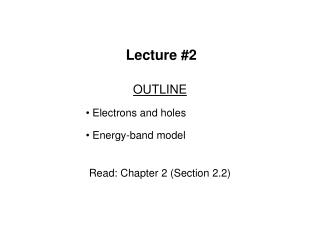DownloadDownload PresentationLecture #2

# Lecture #2

Télécharger la présentation## Lecture #2

- - - - - - - - - - - - - - - - - - - - - - - - - - - E N D - - - - - - - - - - - - - - - - - - - - - - - - - - -
##### Presentation Transcript

1. Lecture #2 OUTLINE Electrons and holes Energy-band model Read: Chapter 2 (Section 2.2)

2. Electronic Properties of Si Silicon is a semiconductor material. • Pure Si has a relatively high electrical resistivity at room temperature.  There are 2 types of mobile charge-carriers in Si: • Conduction electronsare negatively charged; • Holesare positively charged.  The concentration (#/cm3) of conduction electrons & holes in a semiconductor can be modulated in several ways: • by adding special impurity atoms ( dopants ) • by applying an electric field • by changing the temperature • by irradiation EE130 Lecture 2, Slide 2

3. Si Si Si Si Si Si Si Si Si Bond Model of Electrons and Holes 2-D representation: When an electron breaks loose and becomes a conduction electron, a hole is also created. EE130 Lecture 2, Slide 3

4. Mobile positive charge associated with a half-filled covalent bond Treat as positively charged mobile particle in the semiconductor Fluid analogy: What is a Hole? EE130 Lecture 2, Slide 4

5. The Hole as a Positive Mobile Charge EE130 Lecture 2, Slide 5

6. Pure Si conduction ni 1010 cm-3 at room temperature EE130 Lecture 2, Slide 6

7. Definition of Terms n = number of electrons/cm3 p = number of holes/cm3 ni= intrinsic carrier concentration In a pure semiconductor, n = p = ni EE130 Lecture 2, Slide 7

8. Si: From Atom to Crystal Energy states in Si atom  energy bands in Si crystal • The highest nearly-filled band is the valence band • The lowest nearly-empty band is the conduction band EE130 Lecture 2, Slide 8

9. Energy Band Diagram Ec electron energy Ev distance Simplified version of energy band model, indicating • bottom edge of the conduction band (Ec) • top edge of the valence band (Ev) • Ec and Ev are separated by the band gap energy EG EE130 Lecture 2, Slide 9

10. Summary • In a pure Si crystal, conduction electrons and holes are formed in pairs. • Holes can be considered as positively charged mobile particles which exist inside a semiconductor. • Both holes and electrons can conduct current. • Splitting of allowed atomic energy levels occurs in a crystal • Separation between energy levels is small, so we can consider them as bands of continuous energy levels • Highest nearly-filled band is the valence band • Lowest nearly-empty band is the conduction band EE130 Lecture 2, Slide 10

11. Energy-band diagram: • Shows only bottom edge of conduction band Ecand top edge of valence band Ev • Ec and Ev are separated by the band-gap energy EG EE130 Lecture 2, Slide 11